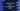# How to print a multiplication table in HTML,CSS, and JavaScript## How to print a multiplication table in HTML,CSS, and JavaScript:

In this post, we will learn how to print a multiplication table in HTML,CSS and JavaScript. Before writing the HTML, CSS part, I will show you how to write it in pure JavaScript. Then we will migrate the code to HTML,CSS and check how it looks like.

### JavaScript program to print a multiplication table:

Let’s write the program first using JavaScript:

``````const number = parseInt(prompt("Enter a number : "));

for (let i = 1; i <= 10; i++) {
console.log(number + "*" + i + "=" + number * i);
}``````

To run this program, open your developer console and paste the above program. It will ask you to enter a number with a popup. Enter the number and it will print the multiplication table for that number.

### Using HTML, CSS with JavaScript:

Let’s use HTML, CSS with JavaScript to print the multiplication table. Create one example.html file and copy paste the below content:

``````<!DOCTYPE html>
<html lang="en">
<style>
button {
background-color: #806ae4;
border: none;
color: white;
text-align: center;
text-decoration: none;
font-size: 15px;
cursor: pointer;
}
p{
color: #27186b;
}
</style>
<script>
var number;
var result = "";

number = Number(document.getElementById("number").value);

for(var i = 1; i<= 11; i++){
result = result + "<p>"+number + "*" + i + "=" + number * i+"</p>";
}

document.getElementById("result").innerHTML = result;
}
</script>
<body>
Enter the number : <input id="number" />
<div id="result">
</body>
</html>``````

Open that file in your browser, it will look as like below:

In this example,

• The style block includes the css styles and the script block JavaScript part.
• Inside body, we have one input that takes the number as input from the user. One button is also there which is used to invoke the addNumbers function in the JavaScript if the user clicks on it. See, how easy it is to run JavaScript from HTML !!
• Inside addNumbers, we are adding all lines of the multiplication table to a variable result. Each line is wrapped in a

tag.
• Once the loop is done, we are getting one element by its id result, which is a
in the body part and changing its innerHTML proparty to set the table in the
.